# Power Spectrum in MATLAB

Posted by in on 4-8-13

One of the most common tasks of an electrical engineer–especially a digital signal processing (DSP) engineer–is to analyze signals in our designs. From analog channel propagation models to digital gates, we need to know how a signal behaves. One particular area of interest is power spectrum.

Power spectrum analysis is typically done in MATLAB using the FFT. The math is fairly straightforward, but getting the power and frequency scaling right can sometimes trip up engineers.

If you need to consider distributed noise power that is normalized and specified in dBm/Hz, then please refer to the article on the Power Spectral Density.

This article will demonstrate how to form a power spectrum in MATLAB using the FFT and cover the following concepts:

1. Mathematical Background
1. Power Axis Scaling in dBm
2. Frequency Axis Scaling
2. Power Spectrum in MATLAB
3. M-Code Discussion

## Mathematical Background

This article will assume that the original time-domain signal, x(t), is a voltage signal, such as a capture from an oscilloscope or analog to digital converter (ADC). This is a good assumption since most signals generated in MATLAB and captured in the lab are typically derived from voltage or are actually voltage signals.

The power spectrum of a signal shows how a signal’s power is distributed throughout the frequency domain. To get the time-domain voltage data to the frequency domain, we use Fast Fourier Transform (FFT).

In order to get the amplitude scaling correct, it is important to keep in mind that the signal power is proportional to the squared absolute value of the voltage. To get the correct quantification of signal power in a frequency, we must take in account the impedance of the system. That makes sense because power is defined as P=V^2/R.

Because electrical engineers typically deal with power in units of dBm, this article will demonstrate the power spectrum in units of dBm. The power spectrum can be found using the following equation: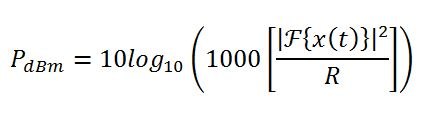where F represents the FFT operation and R is the nominal resistance of system (typically 50 ohms). The scaling factor of 1000 is to place the power in terms of miliwatts so that the final quantity is in dBm after logarithmic scaling.

An important consideration for the discrete signal FFT is that it must be normalized to account for the length of the time-domain signal. The normalized version of the FFT must be used in the power spectrum equation above.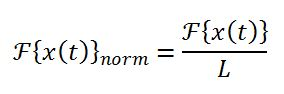where L is the length of the time-domain signal.

The frequency axis is derived from the sampling frequency and the number of points used in the FFT. For MATLAB code shown in this article, we simply use the length of the time-domain signal as the FFT length so that we don’t have to deal with split FFT frequency bins.

The frequency axis extends from -fs/2 to fs/2, with a frequency spacing of fs/nfft, where nfft is the number of FFT points. In this article, we keep the number of FFT points to be the same as the time-domain signal length, L. The frequency axis is limited to |fs/2|, which is the first Nyquist zone where no aliasing occurs.

It’s worth noting that the resolution of the frequency axis is not necessarily a function of the number of FFT points.  While it’s true that number of points on the frequency axis is proportional to the number of FFT points, the true frequency resolution (minimum spacing of frequency components that can be discriminated) is governed by the length of the time domain signal.  This is detailed in both the FFT Frequency Axis and FFT Zero Padding articles.

To provide a sanity check on our answer, we should see peaks in the frequency domain for our sinusoids that follow the equation: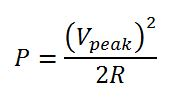For example, a 1 V peak signal should yield 10 dBm of power in a 50 ohm system (note that the equation is in linear units).

## Power Spectrum in MATLAB

[cc lang=”matlab” noborder=”true” tab_size=”4″ lines=”-1″ width=”600″ escaped=”true”]
% system impedance (ohms)
R=50;

% sampling frequency (Hz)
fs=100e6;

% number of time-domain samples
L=1e6;

% time vector for time-domain signal (s)
t=1/fs*[1:L];

% frequency vector for frequency-domain signal (Hz)
nfft=L;
f=fs/2*[-1:2/nfft:1-2/nfft];

% create demonstration sinusoids and noise signals (V)
ns=wgn(L,1,-10,1,[],’dBm’,’real’).’;
s=1*cos(2*pi*18e6*t)+0.02*cos(2*pi*35e6*t)+ns;

% normalized FFT of signal
S=(fftshift(fft(s,nfft))/(L));

% power spectrum
Sp=10*log10((abs(S).^2)/R*1000);

% plot time-domain signal
figure(1)
clf
plot(t/1e-9,s)
title(‘Time-Domain Signal’)
xlabel(‘Time (ns)’)
ylabel(‘Amplitude (V)’)
axis([0 1000 -1 1])

% plot power spectrum
figure(2)
clf
plot(f/1e6,Sp)
title(‘Power Spectrum Using Linear Scale’)
xlabel(‘Frequency (MHz)’)
ylabel(‘Relative Amplitude (linear)’)
[/cc]

## M-Code Discussion

In the example m-code above, I’ve constructed a signal with two sinusoids and some Gaussian noise. The amplitudes for the two sinusoids are 1 V-peak at 18 MHz and 20 mV-peak at 35 MHz, which should yield powers of approximately 10 dBm and -24 dBm respectively.

A portion of the time-domain signal and the full power spectrum are shown in the following figures: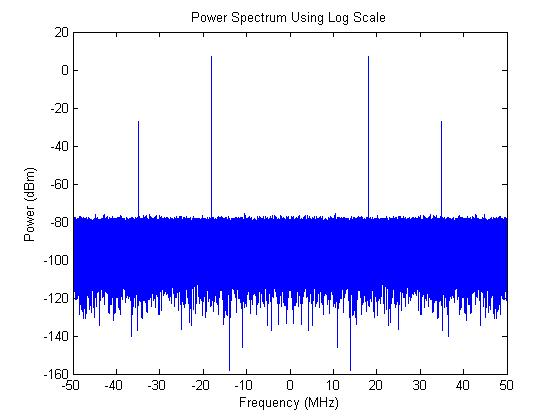If you run the code yourself, you’ll notice that the peaks are located at +/-18 MHz and +/- 35 MHz, which is what we expect. You may be wondering why the peaks do not have an amplitude of 10 dBm and -24 dBm, or why there are 4 peaks instead of 2. This is a consequence of the Fourier Transform, which says that a sinusoid at frequency, f, with amplitude, A, in the time domain will become a pair of peaks located at +/-f with amplitudes of A/2 each.

This means that the two-sided power spectrum peaks will be 3 dB down from the full power of the sinusoid.

Under certain circumstances, a single-sided spectrum can be performed by simply doubling the power and plotting only one side. This can only be done for real-valued signals! For complex signals, the spectrum is not symmetrical, and both sides of the spectrum contain unique information.

You can verify this by replacing the cosines in the code with exp(1i*2*pi*18e6*t) and exp(1i*2*pi*35e6*t). The complex sinusoids only appear in the positive frequency axis. Note that the power has doubled to 13 dBm and -21 dBm because the exponential function consists of two sinusoids.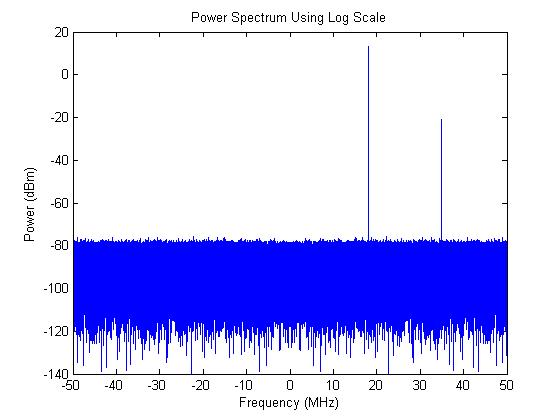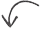Did you enjoy this article?